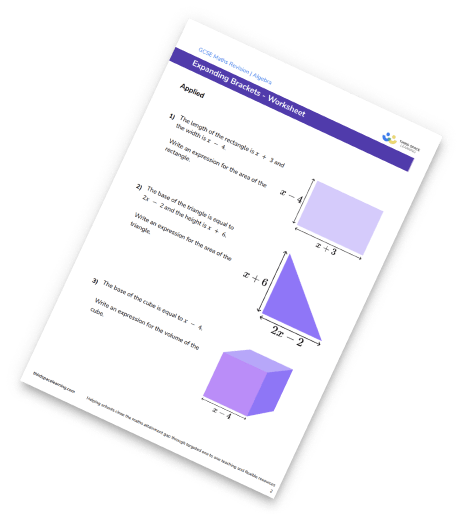# Algebraic Fractions Worksheet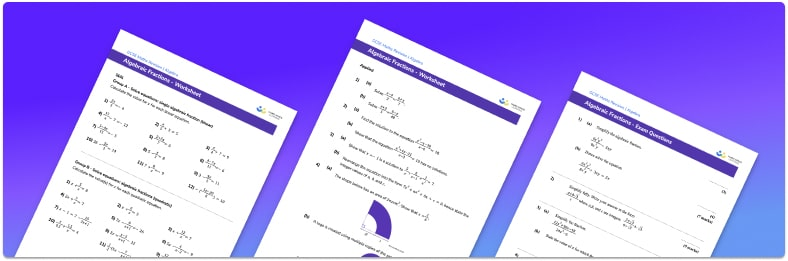• This field is for validation purposes and should be left unchanged.

You can unsubscribe at any time (each email we send will contain an easy way to unsubscribe). To find out more about how we use your data, see our privacy policy.

• Section 1 of the algebraic fractions worksheet contains 36 skills-based factorising questions, in 3 groups to support differentiation
• Section 2 contains 4 applied algebraic fractions questions with a mix of worded problems and deeper problem solving questions
• Section 3 contains 4 foundation and higher level GCSE exam style algebraic fractions questions
• Answers and a mark scheme for all practice questions are provided
• Questions follow variation theory with plenty of opportunities for students to work independently at their own level
• All questions created by fully qualified expert secondary maths teachers
• Suitable for GCSE maths revision for AQA, OCR and Edexcel exam boards

### Algebraic fractions at a glance

Algebraic fractions cover a range of algebraic skills including solving equations, starting with linear equations and moving onto quadratic equations. We can use equivalent fractions to write algebraic fractions in their simplest form by cancelling common factors in the numerator and denominator.

We can also use a common denominator for adding or subtracting algebraic fractions so that they can be written as a single fraction. We can use multiply and dividing algebraic fractions to answer these questions.Looking forward, students can then progress to additional algebra worksheets, for example a straight line graphs worksheet or simultaneous equations worksheet.

For more teaching and learning support on Algebra our GCSE maths lessons provide step by step support and lesson plans for all GCSE maths concepts.

When you have students who require more intensive support our one to one GCSE maths revision programme will match them with the most appropriate tutor. This way we can provide individual students with personalised programmes of study while you continue to teach the rest of your class as a whole group.

Our maths interventions are currently only available for GCSE students, and are not suitable for A level students.

## Related worksheets

Collecting Like Terms Worksheet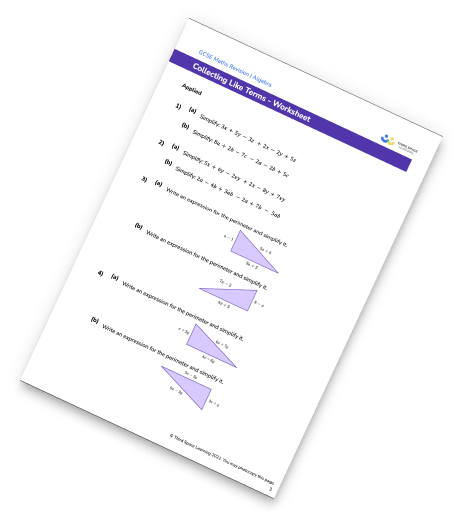Algebraic Expressions Worksheet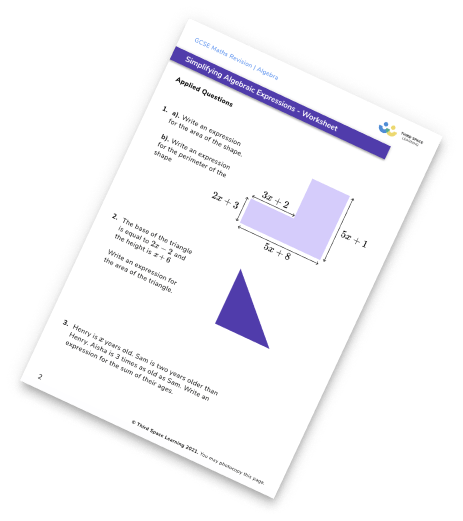Simplifying Algebraic Expressions Worksheet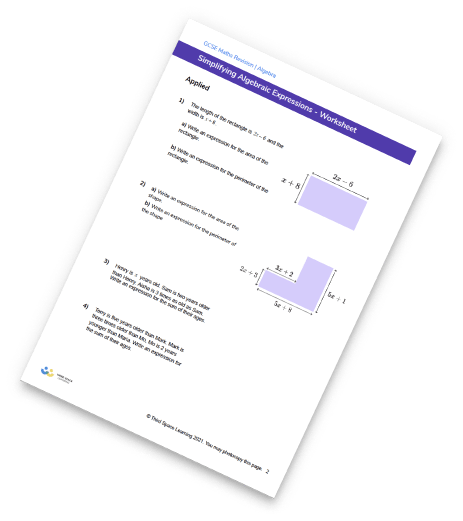Expanding Brackets Worksheet